# Texas Go Math Grade 3 Lesson 7.3 Answer Key Multiply with 3 and 6

Refer to our Texas Go Math Grade 3 Answer Key Pdf to score good marks in the exams. Test yourself by practicing the problems from Texas Go Math Grade 3 Lesson 7.3 Answer Key Multiply with 3 and 6.

## Texas Go Math Grade 3 Lesson 7.3 Answer Key Multiply with 3 and 6

Essential Question
what are some ways to multiply with 3 and 6?

Unlock the Problem
Sabrina is making triangles with toothpicks. She uses 3 toothpicks for each triangle. She makes 4 triangles.
How many toothpicks does Sabrina use?
She uses 3 toothpicks for each triangle.

Explanation:
Number of toothpicks she uses = 3.

• Why does Sabrina need 3 toothpicks for each triangle?
Sabrina need 3 toothpicks for each triangle because to make 4 triangles.

Explanation:
To make 4 triangles Sabrina needs 3 toothpicks for each triangle.

Draw a picture.

Step 1
Complete the 4 triangles.Step 2
Skip count by the number of sides. ___, ___, ___, ___,
How many craft sticks will she use in all? ____
How many toothpicks are there in all?
4 × __ = ___
4 triangles have ___ toothpicks.
So, Sabrina uses ___ toothpicks.

Math Talk
Mathematical Processes
How can you use what you know about the number of toothpicks needed for 4 triangles to find the number of toothpicks needed for 8 triangles? Explain.

Jessica is using craft sticks to make 6 octagons. How many craft sticks will she use in all?Use doubles.
When at least one factor is an even number, you can use doubles.
First multiply with half of an even number.
After you multiply, double the product.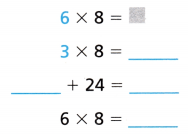Another Way
Use a multiplication table.
Find the product 6 × 8 where row 6 and column 8 meet.
6 × 8 = ___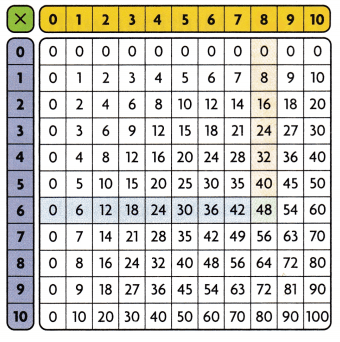• Shade the row for 3 in the table. Then, compare the rows for 3 and 6. What do you notice about their products?
6 × 8 = 48.
It is noticed that 3 products are half of 6. 6 products are double of 3 products.

Explanation:Question 1.
Use 5s facts and addition to find 6 × 4 =___
6 × 4 = 5 × 4 + 4 = 24
6 × 4 = ___6 × 4 = 24.

Explanation:
6 × 4 = 5 × 4 + 4
=> 6 × 4 = 20 + 4
=> 6 × 4 = 24.Math talk
Mathematical Processes
Explain how you would use 5s facts and addition to find 6 × 3.
6 × 3 = 5 × 3 + 3 = 18.
= 15 + 3
= 18.

Explanation:
6 × 3 = 5 × 3 + 3
= 15 + 3
= 18.

Find the product.
Question 2.
6 × 1 = ___
6 × 1 = 6.

Explanation:
6 × 1 =  5 × 1 + 1
= 5 + 1
= 6.

Question 3.
___ = 3 × 7
21 = 3 × 7.

Explanation:
3 × 7 = 2 × 7 + 7
= 14 + 7
= 21.

Question 4.
___ = 6 × 5
30 = 6 × 5.

Explanation:
6 × 5 = 5 × 5 + 5
= 25 + 5
= 30.

Question 5.
3 × 9 = ___
3 × 9 = 27.

Explanation:
3 × 9 = 2 × 9 + 9
= 18 + 9
= 27.

H.O.T. Algebra Complete the table.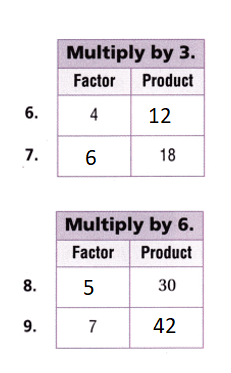Explanation:
6. 4 × 3 = 3 × 3 + 3
= 9 + 3 = 12.
7.  ?? × 3 = 18.
=> ?? = 18 ÷ 3
=> ?? = 6.
8. ?? × 6 = 30.
=> ?? = 30 ÷ 6
=> ?? = 5.
9. 7 × 6 = 6 × 6 + 6
=> 36 + 6 = 42.

Problem Solving
Use the table for 12-13.
Question 12.
Use Tools The table tells about quilt pieces Jenna has made. How many squares are there in 6 of Jenna’s quilt pieces?Number of squares in 6 of Jenna’s quilt pieces = 36.

Explanation:
Number of squares in one quilt pieces = 6.
Number of quilt pieces Jenna’s having = 6.
Number of squares in 6 of Jenna’s quilt pieces = Number of squares in one quilt pieces × Number of quilt pieces Jenna’s having
= 6 × 6
= 5 × 6 + 6
= 30 + 6
= 36.

Question 13.
Compare How many more squares than triangles are in 3 of Jenna’s quilt pieces?
3 more squares than triangles are in 3 of Jenna’s quilt pieces.

Explanation:
Number of squares in one quilt pieces = 6.
Number of quilt pieces Jenna’s having = 3.
Number of squares in 3 of Jenna’s quilt pieces = Number of squares in one quilt pieces × Number of quilt pieces Jenna’s having
= 6 × 3
= 5 × 3 + 3
= 15 + 3
= 18.
Number of triangles in one quilt pieces = 4.
Number of quilt pieces Jenna’s having = 3.
Number of triangles in 3 of Jenna’s quilt pieces = Number of triangles in one quilt pieces × Number of quilt pieces Jenna’s having
= 4 × 3
= 3 × 3 + 3
= 9 + 3
= 12.
Difference:
Number of squares in 3 of Jenna’s quilt pieces – Number of triangles in 3 of Jenna’s quilt pieces
= 18 – 15
= 3.

Question 14.
H.O.T. Multi-Step Alii had some craft sticks. She decided to make shapes. If she used one craft stick for each side of the shape, would Alii use more craft sticks for 5 squares or 6 triangles? Explain.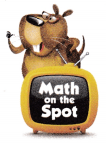Alii use 2 more craft sticks for 5 squares than 6 triangles.

Explanation:
Number of craft stick for each side of the shape she used = 1.
Number of sides for a square = 4.
Number of craft sticks for making 5 squares = 5 × (Number of craft stick for each side of the shape she used × Number of sides for a square)
= 5 × (1 × 4)
= 5 × 4
= 4 × 4 + 4
= 16 + 4
= 20.
Number of sides for a triangle = 3.
Number of craft sticks for making 6 triangle = 6 × (Number of craft stick for each side of the shape she used × Number of sides for a triangle)
= 6 × (1 × 3)
= 6 × 3
= 18.
Difference:
Number of craft sticks for making 5 squares – Number of craft sticks for making 6 triangle
= 20 – 18
= 2.

Question 15.
H.O.T. Multi-Step Draw a picture and use words to explain the Commutative Property of Multiplication with the factors 3 and 4.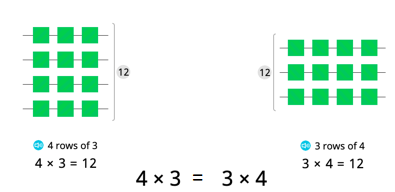Explanation:
The commutative property states that the change in the order of numbers in an addition or multiplication operation does not change the sum or the product. The commutative property of addition is written as A + B = B + A. The commutative property of multiplication is written as A × B = B × A.
Suppose: 3 × 4 = 12.
4 × 3 = 12.
=> 3 × 4 = 4 × 3.

Fill in the bubble for the correct answer choice.
Use multiplication strategies to solve.
Question 16.
Each day a hornworm eats 8 leaves. How many leaves will the hornworm eat in 3 days?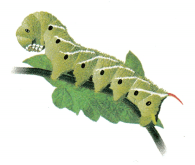(A) 21
(B) 11
(C) 24
(D) 16
Number of leaves will the hornworm eat in 3 days = 24.
(C) 24.

Explanation:
Number of leaves each day a hornworm eats = 8.
Number of leaves will the hornworm eat in 3 days = 3 × Number of leaves each day a hornworm eats
= 3 × 8
= 2 × 8 + 8
= 16 + 8
= 24.

Question 17.
Representation Which shows a way to use a 5s fact to find 6 × 9?
(A) 5 × 9 + 9
(B) 5 × 9 + 5
(C) 5 × 5 + 5
(D) 5 × 9 + 6
5s fact to find 6 × 9: 6 × 9 = 5 × 9 + 9 = 54.
(A) 5 × 9 + 9

Explanation:
5s fact to find 6 × 9:
6 × 9 = 5 × 9 + 9
= 45 + 9
= 54.

Question 18.
Multi-Step Bobbie has 4 packs of trading cards. Each pack holds 6 cards. Bobbie gives 6 of the cards to his sister. How many cards does Bobbie have left?
(A) 2
(B) 24
(C) 18
(D) 36
Number of cards Bobbie have left = 18.
(C) 18.

Explanation:
Number of packs of trading cards Bobbie has = 4.
Number of cards each pack holds = 6.
Number of cards Bobbie gives to his sister = 6.
Number of cards Bobbie have left = (Number of packs of trading cards Bobbie has × Number of cards each pack holds) – Number of cards Bobbie gives to his sister
= (4 × 6) – 6
= 24 – 6
= 18.

Texas Test Prep
Question 19.
There are 8 crayons in each of 6 packages. How many crayons are there?
(A) 24
(B) 14
(C) 2
(D) 48
Number of crayons are there = 48.
(D) 48.

Explanation:
Number of crayons in each pack = 8.
Number of packages of crayons = 6.
Number of crayons are = Number of crayons in each pack × Number of packages of crayons
= 8 × 6
= 7 × 6 + 6
= 42 + 6
= 48.

### Texas Go Math Grade 3 Lesson 7.3 Homework and Practice Answer Key

Algebra Complete the table.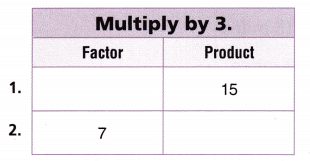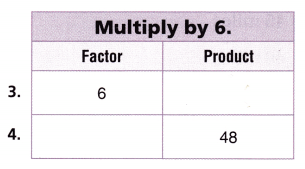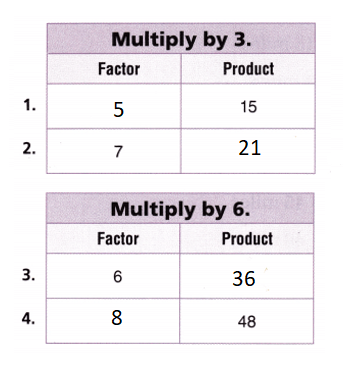Explanation:
Multiple by 3:
1. ?? × 3 = 15.
=> ?? = 15 ÷ 3
=> ?? = 5.
2. 7 × 3 = 21.
Multiple by 6:
3. 6 × 6 = 36.
4. ?? × 6 = 48.
=> ?? = 48 ÷ 6
=> ?? = 8.

Use the table for 5-8.
Craft Stick Animals
Question 5.
Joella makes 6 craft stick birds. How many craft sticks does Joella use?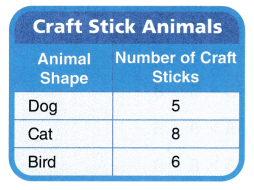Number of craft sticks Joella uses = 36.

Explanation:
Number of craft stick birds Joella makes = 6.
Number of birds craft sticks = 6.
Number of craft sticks Joella uses = Number of craft stick birds Joella makes × Number of birds craft sticks
= 6 × 6
= 5 × 6 + 6
= 30 + 6
= 36.

Question 6.
Lebraun makes 3 dogs and 2 birds. Does he use more craft sticks for the birds or for the dogs?
He uses 3 more craft sticks for the birds than for the dogs.

Explanation:
Number of dogs Lebraun makes = 3.
Number of dog craft sticks = 5.
Number of craft stick dogs Lebraun makes = Number of dogs Lebraun makes × Number of dog craft sticks
= 3 × 5
= 2 × 5 + 5
= 10 + 5
= 15.
Number of birds Lebraun makes = 2.
Number of birds craft sticks = 6.
Number of craft stick birds Lebraun makes = Number of birds Lebraun makes × Number of birds craft sticks
= 2 × 6
= 1 × 6 + 6
= 6 + 6
= 12.
Difference:
Number of craft stick dogs Lebraun makes – Number of craft stick birds Lebraun makes
= 15 – 12
= 3.

Question 7.
Ariel makes 2 cats and 2 dogs. How many more craft sticks does she need to make the cats?
6 more craft sticks she needs to make the cats.

Explanation:
Number of dogs Ariel makes = 2.
Number of dog craft sticks = 5.
Number of craft stick dogs Ariel makes = Number of dogs Ariel makes × Number of dog craft sticks
= 2 × 5
= 1 × 5 + 5
= 5 + 5
= 10.
Number of cats Ariel makes = 2.
Number of cats craft sticks = 8.
Number of craft stick cats Ariel makes = Number of cats Ariel makes × Number of cats craft sticks
= 2 × 8
= 1 × 8 + 8
= 8 + 8
= 16.
Difference:
Number of craft stick cats Ariel makes – Number of craft stick dogs Ariel makes
= 16 – 10
= 6.

Problem Solving
Question 8.
Sam makes 3 dogs and 2 cats. Does he need more craft sticks for the dogs or for the cats? Explain.
He need 1 more craft sticks for the cats than for the dogs.

Explanation:
Number of dogs Sam makes = 3.
Number of dog craft sticks = 5.
Number of craft stick dogs Sam makes = Number of dogs Sam makes × Number of dog craft sticks
= 3 × 5
= 2 × 5 + 5
= 10 + 5
= 15.
Number of cats Sam makes = 2.
Number of cats craft sticks = 8.
Number of craft stick cats Sam makes = Number of cats Sam makes × Number of cats craft sticks
= 2 × 8
= 1 × 8 + 8
= 8 + 8
= 16.
Difference:
Number of craft stick cats Sam makes – Number of craft stick dogs Sam makes
= 16 – 15
= 1.

Lesson Check
Question 9.
Each day Mr. Mosell drives a total of 8 miles to work and back. How many miles does Mr. Mosell drive in 5 days?
(A) 45 miles
(B) 40 miles
(C) 13 miles
(D) 58 miles
Number of miles Mr. Mosell drives in 5 days = 40.
(B) 40 miles.

Explanation:
Number of miles each day Mr. Mosell drives to work and back = 8.
Number of miles Mr. Mosell drives in 5 days = 5 × Number of miles each day Mr. Mosell drives to work and back
= 5 × 8
= 4 × 8 + 8
= 32 + 8
= 40.

Question 10.
Tanya walks 3 miles every day for one week. How many miles does Tanya walk in all?
(A) 7 miles
(B) 15 miles
(C) 3 miles
(D) 21 miles
Number of miles Tanya walk in all = 21.
(D) 21 miles.

Explanation:
Number of miles every day for one week Tanya walks = 3.
Number of days in a week = 7.
Number of miles Tanya walk in all = Number of miles every day for one week Tanya walks × Number of days in a week
= 3 × 7
= 2 × 7 + 7
= 14 + 7
= 21.

Question 11.
Terrel wants to use a 5s fact to multiply 6 × 8. Which expression can Terrel use?
(A) 5 × 5 + 8
(B) 5 × 8 + 5
(C) 5 × 8 + 8
(D) 6 × 8 + 5
Expression can Terrel use is 6 × 8 = 5 × 8 + 8 = 48.
(C) 5 × 8 + 8.

Explanation:
Terrel wants to use a 5s fact to multiply 6 × 8:
=> 6 × 8 = 5 × 8 + 8
=> 6 × 8 = 40 + 8
=> 6 × 8 = 48.

Question 12.
Anita wants to use a 5s fact to multiply 6 × 3. Which expression can Anita use?
(A) 5 × 3 + 3
(B) 5 × 3 + 5
(C) 5 × 8 + 5
(D) 5 × 3 + 6
Expression can Anita use is 6 × 3 = 5 × 3 + 3 = 18.
(A) 5 × 3 + 3.

Explanation:
Anita wants to use a 5s fact to multiply 6 × 3:
=> 6 × 3 = 5 × 3 + 3
=> 6 × 3 = 15 + 3
=> 6 × 3 = 18.

Question 13.
Multi-Step Milo collects 6 boxes of shells. Each box holds 7 shells. Milo gives 2 boxes of shells to his friend. How many shells does Milo have left?
(A) 30
(B) 42
(C) 14
(D) 28
Number of shells Milo have left = 28.
(D) 28.

Explanation:
Number of boxes of shells Milo collects = 6.
Number of shells each box holds = 7.
Number of boxes of shells Milo gives to his friend = 2.
Number of shells Milo have left = (Number of boxes of shells Milo collects × Number of shells each box holds) – (Number of boxes of shells Milo gives to his friend × Number of shells each box holds)
= (6 × 7) – (2 × 7)
= 42 – 14
= 28.

Question 14.
Multi-Step Henley collects 3 eggs from each of the nests of 9 chickens. Henley uses 6 eggs to make breakfast. How many eggs does Henley have left?
(A) 21
(B) 27
(C) 18
(D) 54